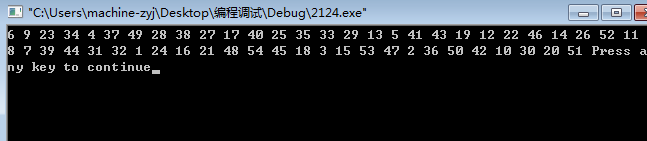# 代码

//////////  QQ斗地主发牌
#include<iostream>
#include <ctime>
using namespace std;
int num;
int in(int temp,int count){
for(int i=0;i<count){
if(num[i]==temp) return 0;
}
return 1;
}
int main(){
int count=0,temp;
srand(time(NULL));
while(count<=54){
temp = rand()%54+1;
if(in(temp,count)){
num[count]=temp;
count++;
}
}
for(int i=0;i<54;i++){
cout<<num[i]<<" ";
}
}